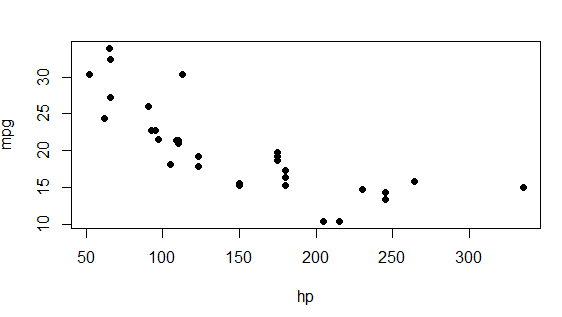2 views

In R, the plot() function takes a pch argument that controls the appearance of the points in the plot. I'm making scatterplots with tens of thousands of points and prefer a small, but not too small dot. Basically, I find pch='.' to be too small, but pch=19 to be too fat. Is there something in the middle or some way to scale the dots down somehow?

by

To change the size of the points in R scatterplot, you can use the cex argument.

According to R Documentation:

cex

A numerical value giving the amount by which plotting text and symbols should be magnified relative to the default. This starts at 1 when a device is opened and is reset when the layout is changed, e.g. by setting mfrow.

cex.axis

The magnification to be used for axis annotation relative to the current setting of cex.

cex.lab

The magnification to be used for x and y labels relative to the current setting of cex.

cex.main

The magnification to be used for main titles relative to the current setting of cex.

cex.sub

The magnification to be used for subtitles relative to the current setting of cex

For example:

data(mtcars)

plot(mpg ~ hp, data = mtcars, pch = 20, cex  = 1.5)

pch = 20:- bullet (smaller solid circle, 2/3 the size of 19),

cex =1.5 :- To magnify to 150 percent.

Output: